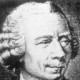## Puzzler from Car Talk

byAny of you listen to Car Talk, the auto show by Tom and Ray?  It’s a great weekend listen (although thanks to the glory of the Internet, you can hear episodes at pretty much any time from pretty much any place with an internet connection).  During each show they have a Puzzler, the answer to which they reveal the following week.  Some of the puzzlers have a mathematical bent, like the one from Ray for the week of December 8:

This puzzler is from my mathematical series. Every two-digit number can be represented as AB, where B is the ones digit and A is the tens digit. Right? So for example the number 43, A is 4 and B is 3.

Imagine then that you took this two-digit number and you squared it, AB x AB, and when you did that the result was a three-digit number, CAB.

Here’s the question: What’s the value of C? So, for example if AB is 43, CAB might be 943. Of course this is a totally bogus answer, but you get the idea.

So again, what is the value of C, so that AB(squared)= CAB?

This can be done by brute force, but examples of more elegant solutions are certainly welcome!  (Indeed, since the answer has already been posted online, there’s no harm is sharing partial or complete solutions).

Thanks to Ted for bringing this puzzler to my attention!

### 6 Responses to “Puzzler from Car Talk”

1.David P Says:

Here’s what I’ve got so far (then called to family dinner, so it’s left partial)

BxB = ZB
B*A+Z + A*B = 2AB + Z = YA
A*A + Y = C

B = 1, 5, 6
then z = 0, 2, 3 respectively

A*A < 10, so A = 1, 2, 3

2.L. Zoel Says:

x^2-x=n*100 for n={1,2,3,…,9}
Solved them all until you get x an integer.
Not sure if this counts as brute force or not…

3.TwoPi Says:

Since$n\cdot 100 = x(x-1)$, and gcd(x, x-1) = 1, we know that 4 divides either x or x-1, 25 divides either x or x-1, and that x < 100.

Considering multiples of 25, we can eliminate x = 50, since if x is even, then 4 must divide x.

We can eliminate x = 75, since if x is odd, then 4 must divide x-1.

Thus x = 25, and indeed 25*24 = 600. (and 25^2 = 625.)

4.jd2718 Says:

n = n^2 (mod100) so
n = n^2 (mod10) and
n = n^2 (mod4)

I think that’s enough. Last digit 1,5,6
The mod4 requirement kills the 6s.

n^2 l.t. 1000, so n l.t. 32

Only 5 candidates to check

Jonathan

5.Ξ Says:

What I find intriguing is how many different ways there are to solve this problem. My initial approach was to look at A and realize that A could only be 0 [maybe], 1, 2, or 3 since 40*40 gives a 4-digit number.

(Not that that method really panned out, but it was still where my mind first headed.)

6.Joel Neely Says:

40*40 = 1600, so A must be 1, 2, or 3.
B*B must end in B, so B must be 0, 1, 5, or 6.
(A*10) squares to (A*A)*100, so B cannot equal 0.
32*32 = 1024, so if A is 3, B can only equal 1; 31*31 = 961, so A can’t be 3.
That leaves 11, 15, 16, 21, 25, and 26 as candidates, whose squares are 121, 225, 256, 441, 625… Aha!